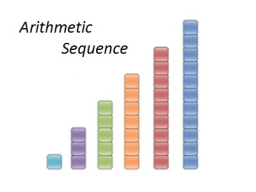# AP five members

Give the arithmetic sequence of 5 terms if the first term is 8 and the last term is 100. Show your solution.

a1 =  8
a2 =  31
a3 =  54
a4 =  77
a5 =  100

### Step-by-step explanation:Did you find an error or inaccuracy? Feel free to write us. Thank you!

Tips for related online calculators
Need help calculating sum, simplifying, or multiplying fractions? Try our fraction calculator.
Do you have a linear equation or system of equations and looking for its solution? Or do you have a quadratic equation?